# SpinnerCube - Rubik's cube solver robot -18 seconds

Using the legacy of brilliant mathematicians, this robot solve the cube in 18 seconds or less.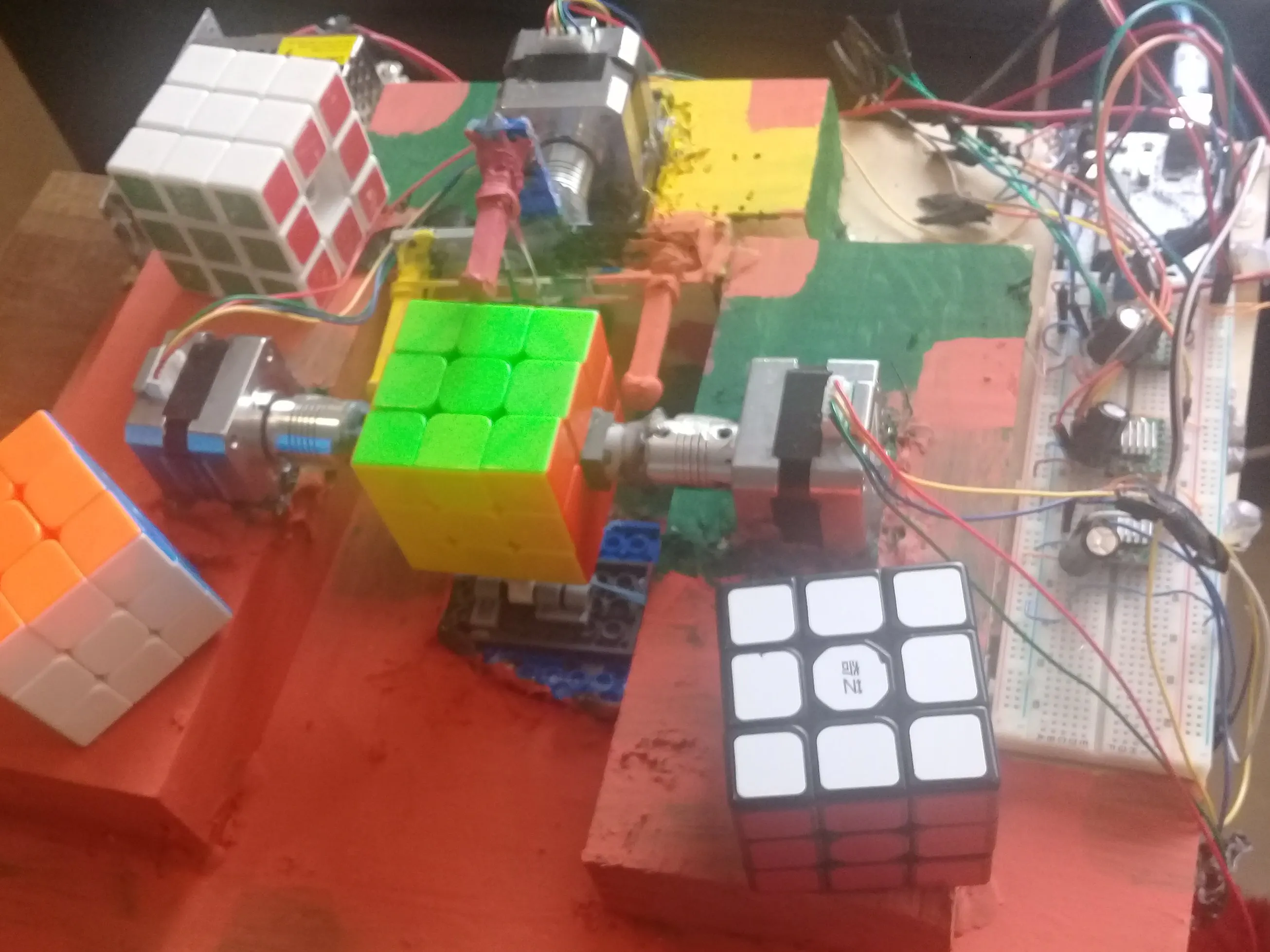## Things used in this project

### Hardware components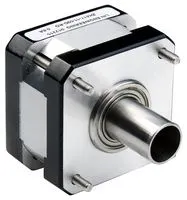Stepper Motor, Bipolar Nema 17
×3Arduino UNO
×1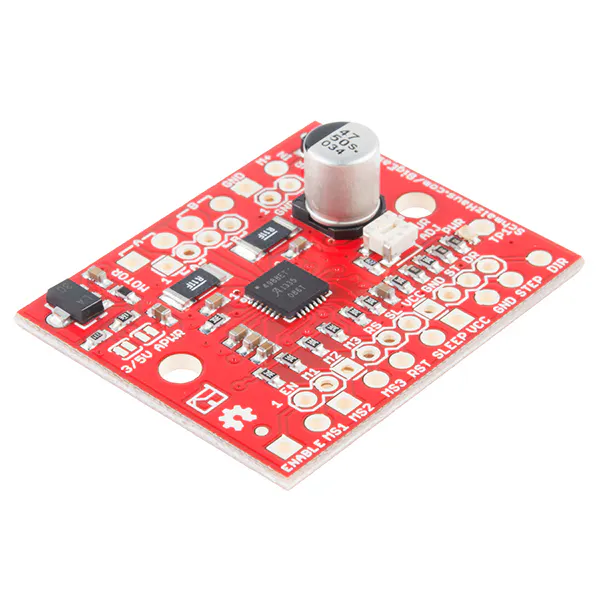SparkFun Stepper motor driver board A4988
×3
 shatf coupler flexible
×3Jumper wires (generic)
×1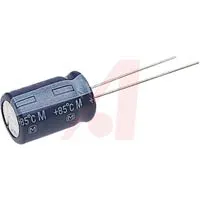Capacitor 10 µF
×3

### Hand tools and fabrication machinesWire Stripper & Cutter, 32-20 AWG / 0.05-0.5mm² Solid & Stranded Wires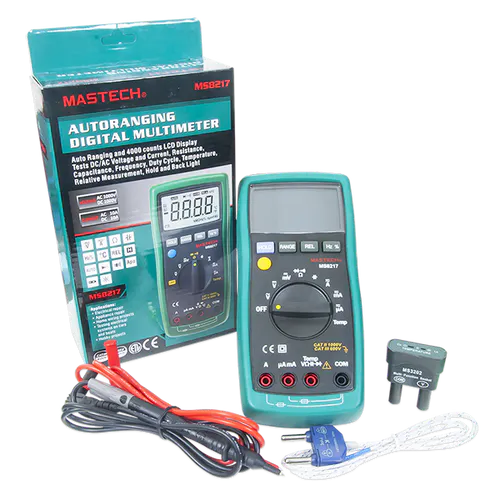Digilent Mastech MS8217 Autorange Digital Multimeter

## Schematics

### skecth_79AeZAyw6s.jpg

Circuit Diagram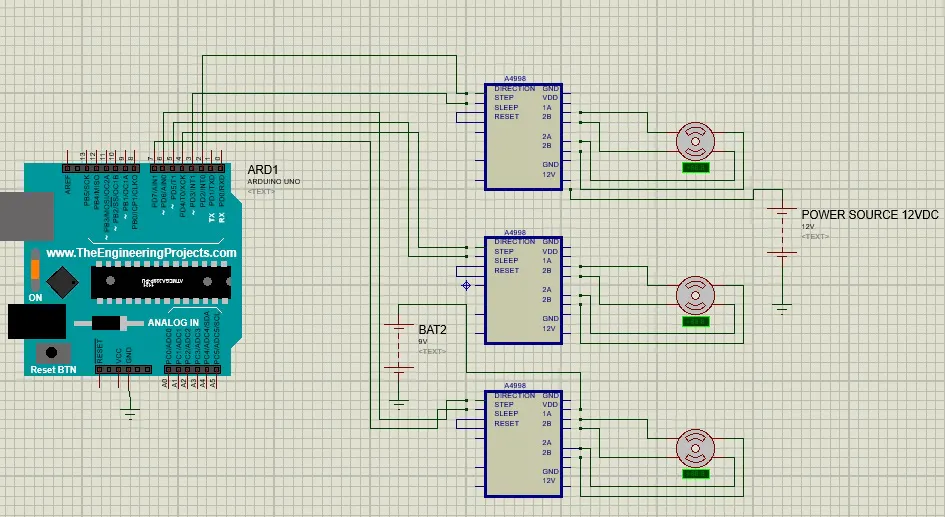## Code

### Arduino Code

Arduino
Receive from Python the solution and run the moves
```/*
*  by hernando Bolaños
*
*  Piece of code from, Matt2Yu, VER instructables
*/

//////////////////////////////////////////////////////////////////////////////////////////////////////
////WARNING: PROTOTYPE BASED ON CUBESPINNER ////
/////////////////////////////////////////////////////////////////////////////////////////////////////

#define STEP_L 4      // pin STEP de A4988 a pin 4 PWM- manejando la cara down del cubo
#define DIR_L 5     // pin DIR de A4988 a pin 5  PWM-manejandola cara down del cubo
#define STEP_R 6      // pin STEP de A4988 a pin 4 PWM-manejando la cara right del cubo
#define DIR_R 7     // pin DIR de A4988 a pin 5  PWM-manejando la cara righ delcubo

#define STEP_F 8      // pin STEP de A4988 a pin 4- manejando la cara "up" del cubo
#define DIR_F 9     // pin DIR de A4988 a pin 5  -manejandola cara "up" del cubo

int pasos = 200/4;    // LATERALES pasos   90 grados con motor Hanpose 17HS3430 : 1.8 grados por paso en condicion Full step- Se probo comportamiento en otras configuraciones de pasos
int Fpasos1=274/4;    //FRENTE Pasos para angulo de front avanzar la cara
int Fpasos2=74/4;    //FRENTE Pasos para angulo de front quedar en posicion esperando nuevo movimiento
int Fpasos3=268/4;    //FRENTE Pasos para angulo de front inv quedar en posicion esperando nuevo movimiento-front inv
int Fpasos4=70/4;     //FRENTE Pasos para angulo de front inv quedar en posicion esperando nuevo movimiento-front inv
int pasosr=3*pasos;
String kociemba_sol = "";

int tiempo_step= 600; //microseconds -segun DS minimo tiempo permitido del step de motores 1 microsegundo
int tiempo_stepF= 1400; //microseconds- tiempo para usar en el frente con los demas andando en tiempo_step

int espera=200; //tiempo de espera despues de un movimiento

int esperaT=50;

void setup()
{

pinMode(STEP_L, OUTPUT);  // pin 4 como salida
pinMode(DIR_L, OUTPUT);   // pin 5 como salida

pinMode(STEP_R, OUTPUT);  // pin 6 como salida
pinMode(DIR_R, OUTPUT);   // pin 7 como salida

pinMode(STEP_F, OUTPUT);  // pin 8 como salida
pinMode(DIR_F, OUTPUT);   // pin 9 como salida

delay(7000);

Serial.begin(9600);

while (! Serial); // Wait untilSerial is ready
//assign_to_current(yellow_side);
//print_cube(current_side);

}

void loop()
{

//recibir la solucion de kociemba por puerto serial

//accept_string();

//calentando 5 segundos

Serial.println("Calentando para fotos: volvera a la posicion inicial");

//right();
//right_inv();
//front();
//front_inv();
//left();
//left_inv();

delay(2000);

Serial.println("Arduino dice:Inicia a correr la solucion:");

run_kociemba(); //corre el string recibido

Serial.println("Arduino dice: Finaliza tiempo solucion");

//mostrar el cubo resuelto

show_off_cube();

Serial.println("Arduino dice:Finalizado enviar nueva solucion de Kociemba");

while(true){}
}

void accept_string()   ////Se comunica el Arduino con el PC le confirma que esta listo para recibir la solucion en un STRING-la recibe/////
{

for (int piece_num = 0; piece_num <5; piece_num++)
{
delay(100);
}
while(kociemba_sol == "")
{
char character;
while(Serial.available())
{
kociemba_sol.concat(character);
}
}
delay(10);
Serial.print(kociemba_sol);

// Se envia confirmacion de recibido al PC con el STRING para reconfirmar
Serial.println("arduino dice:");
Serial.println(kociemba_sol);
delay(10);
}

////////////////////////////////////////////////////////////////////////////////////////////////////////////////
////Aqui se recibe de python la solucion de kociemba y se convierte en movimientos de los stepper motors"///////
////////////////////////////////////////////////////////////////////////////////////////////////////////////////

void run_kociemba(){

//steppers en posicion inicial- home

kociemba_sol="U B U' RR D L' D F' L U F LL U LL BB U' BB U' FF RR LL";

//Solved from Python for test

//"U B U' RR D L' D F' L U F LL U LL BB U' BB U' FF RR LL";

//"L' U B' DD L DD F' R D R L' UU FF LL BB RR U RR LL FF D";

//"RR FF U' L UU BB D B' D R' F U RR U FF U' LL BB RR LL";

//"R F' B' L' U D' BB R' DD R' F' BB LL FF DD BB U BB RR U DD";

//"FF B' UU RR UU B D' R' F' L' U' BB UU LL D' FF U' FF BB D'";

//"D R FF BB U RR LL B' R D F LL BB LL DD FF D FF BB LL D";
//"R D' LL BB U F BB U F U RR U LL FF U' FF UU D' RR":

//" FFFFBRRRRRRRR'R'R' RRRRLLLLLLLL'L'";

//kociemba_sol= "F'F'F'F'F'FFFFFFFFFFFFFF";

//kociemba_sol="U L' U DD B DD FF B' L' U' B' U' LL U LL U' RR UU RR BB U'";

//"RR U RR B' U F' D BB U' B L' DD RR UU D FF D LL U' FF BB";

//"U BB L U' F' L' B UU R' D' B' D RR U DD BB RR D LL FF D";

//"U F' BB U' F UU F R' U' LL F' L U RR U FF RR UU RR BB DD RR";

//"U R BB DD F' U' F' R F' B' U B DD RR FF U' RR U' BB DD RR FF";

//"FF UU F LL D BB U' RR F' R' U D BB D FF LL D' RR UU LL";

//"UU D' BB DD F' R L F' BB U' L' D' BB U BB LL U FF LL BB UU";

//"U R UU B U' F' U FF BB RR U L U DD LL UU RR D' FF RR D LL";

//"D' RR DD B' RR L' FF D' FF R' D FF RR FF LL BB DD BB D BB";

//"R' D' R' D' F U R BB D' FF R' D FF RR FF LL BB DD BB D BB";

//"F R F L B' RR F' LL D' F L FF UU LL D' LL BB UU FF";

//"B' D' L' U F RR LL F L' F' U' RR U' FF RR LL FF DD FF U'";

//"F' RR U L' U F' D' RR U' DD F' U' BB RR FF U LL U' BB LL";
//"RRRRR'RFBLlU'RLFD'F L BB U RR FF UU LL BB RR DD RR";

// Length (with one extra character for the null terminator)

int str_len = kociemba_sol.length() + 1;

Serial.println("Arduino dice: Caracteres:");
Serial.println((str_len-1));

for(int i = 0; i <= (str_len-1); i++){     //recorre

//Serial.print(i);

if ((kociemba_sol.charAt(i)) =='R'){

if ((kociemba_sol.charAt(i+1)) == '2') {
right();
right();}

if ((kociemba_sol.charAt(i+1)) == '\'') {
right_inv();}

if (((kociemba_sol.charAt(i+1))!= '2') and((kociemba_sol.charAt(i+1)) != '\'')) {
right();}

}

}

if ((kociemba_sol.charAt(i)) =='L'){

if ((kociemba_sol.charAt(i+1)) == '2') {
left();
left();}

if ((kociemba_sol.charAt(i+1)) == '\'') {
left_inv();}

if (((kociemba_sol.charAt(i+1))!= '2') and((kociemba_sol.charAt(i+1)) != '\'')) {
left();}

}

}

if ((kociemba_sol.charAt(i)) =='U'){

if ((kociemba_sol.charAt(i+1)) == '2') {
up();
up();}

if ((kociemba_sol.charAt(i+1)) == '\'') {
up_inv();}

if (((kociemba_sol.charAt(i+1))!= '2') and((kociemba_sol.charAt(i+1)) != '\'')) {
up();}

}

}

if ((kociemba_sol.charAt(i)) =='D'){

if ((kociemba_sol.charAt(i+1)) == '2') {
down();
down();}

if ((kociemba_sol.charAt(i+1)) == '\'') {
down_inv();}

if (((kociemba_sol.charAt(i+1))!= '2') and((kociemba_sol.charAt(i+1)) != '\'')) {
down();}

}

}

if ((kociemba_sol.charAt(i)) == 'F'){

if ((kociemba_sol.charAt(i+1)) == '2') {
front();
front();}

if ((kociemba_sol.charAt(i+1)) == '\'') {
front_inv();}

if (((kociemba_sol.charAt(i+1))!= '2') and((kociemba_sol.charAt(i+1)) != '\'')) {
front();}

}

}

if ((kociemba_sol.charAt(i)) =='B'){

if ((kociemba_sol.charAt(i+1)) == '2') {
back();
back();}

if ((kociemba_sol.charAt(i+1)) == '\'') {
back_inv();}

if (((kociemba_sol.charAt(i+1))!= '2') and((kociemba_sol.charAt(i+1)) != '\'')) {
back();}

}

}

}

}
////////////////////////////////////////////////////////////////////////////
/////Rutinas del Cubo- se puede usar asi : YELLOW at FRONT -BLUE at UP//////
///////////////////////////////////////////////////////////////////////////

void right_inv()// movimiento RIGHT inverso del Cubo
{

Serial.println("R',");

digitalWrite(DIR_R, LOW);    //
for(int i = 0; i < pasosr; i++){     //Mueve 3x90 grados
digitalWrite(STEP_R, HIGH);       // nivel alto
delayMicroseconds(tiempo_step);
delayMicroseconds(tiempo_step);// por x ms segun
digitalWrite(STEP_R, LOW);        // nivel bajo variable tiempo_step
delayMicroseconds(tiempo_step);          // por x ms segun variable tiempo_step
delayMicroseconds(tiempo_step);
}
delay(espera);

}

void right()// mueve el cubo Right
{

Serial.println("R,");

digitalWrite(DIR_R, LOW);     //
for(int i = 0; i < pasos; i++){   //
digitalWrite(STEP_R, HIGH);
delayMicroseconds(tiempo_step);
delayMicroseconds(tiempo_step);
digitalWrite(STEP_R, LOW);
delayMicroseconds(tiempo_step);
delayMicroseconds(tiempo_step);
}
delay(espera);          // demora de x segundos

}

void up()// mueve el cubo UP
{

Serial.println("U,");

rinv_l();
delay(100);
front();
delay(100);
rinv_l();
rinv_l();
rinv_l();
delay(espera);
}

void up_inv()// mueve el cubo  UP INV
{

Serial.println("U',");

rinv_l();
delay(100);
front_inv();
delay(100);
rinv_l();
rinv_l();
rinv_l();
delay(espera);

}

void down()// mueve el cubo UP
{

Serial.println("D,");

rinv_l();
rinv_l();
rinv_l();
delay(100);
front();
delay(100);
rinv_l();
delay(espera);

}

void down_inv()// mueve el cubo Down_INV
{

Serial.println("D',");

rinv_l();
rinv_l();
rinv_l();
delay(100);
front_inv();
delay(100);
rinv_l();
delay(espera);

}

void left()// mueve el cubo LEFT

{

Serial.println("L,");

digitalWrite(DIR_L, LOW);    // giro en  sentido
for(int i = 0; i < pasosr; i++){     //
digitalWrite(STEP_L, HIGH);       // nivel alto
delayMicroseconds(tiempo_step);          // por x mseg
delayMicroseconds(tiempo_step);
digitalWrite(STEP_L, LOW);        // nivel bajo
delayMicroseconds(tiempo_step);          // por x mseg
delayMicroseconds(tiempo_step);
}
delay(espera);

}

void left_inv()// mueve el cubo LEFT INV
{

Serial.println("L',");

digitalWrite(DIR_L, LOW);     // giro en sentido NCW
for(int i = 0; i < pasos; i++){   //90 grados= xx pasos para motor de yy grados de angulo de paso
digitalWrite(STEP_L, HIGH);
delayMicroseconds(tiempo_step);
delayMicroseconds(tiempo_step);
digitalWrite(STEP_L, LOW);
delayMicroseconds(tiempo_step);
delayMicroseconds(tiempo_step);

}

delay(espera);          // demora de 2 segundos
}

void front_inv()// mueve el cubo front_inv
{
Serial.println("F',");

digitalWrite(DIR_F, HIGH);    // giro en  sentido CW
for(int i = 0; i < Fpasos3; i++){     //135 grados= xx pasos para motor de yy grados de angulo de paso
digitalWrite(STEP_F, HIGH);       // nivel alto
delayMicroseconds(tiempo_stepF);          // por 10 mseg
digitalWrite(STEP_F, LOW);        // nivel bajo
delayMicroseconds(tiempo_stepF);          // por 10 mseg
}

delay(100);

digitalWrite(DIR_F, LOW);
for(int i = 0; i < Fpasos4; i++){     //45 grados= 25x16 pasos para motor de 1.8 grados de angulo de paso
digitalWrite(STEP_F, HIGH);       // nivel alto
delayMicroseconds(tiempo_stepF);          // por 10 mseg
digitalWrite(STEP_F, LOW);        // nivel bajo
delayMicroseconds(tiempo_stepF);          // por 10 mseg
}
delay(espera);

}

void front()// mueve el cubo  front
{

Serial.println("F,");

digitalWrite(DIR_F, LOW);     //
for(int i = 0; i < Fpasos1; i++){   //135 grados= 70x16 pasos para motor de 1.8 grados de angulo de paso
digitalWrite(STEP_F, HIGH);
delayMicroseconds(tiempo_stepF);
digitalWrite(STEP_F, LOW);
delayMicroseconds(tiempo_stepF);
}

delay(100);
digitalWrite(DIR_F, HIGH);     //
for(int i = 0; i < Fpasos2; i++){   //45 grados= 25x16 pasos para motor de 1.8 grados de angulo de paso
digitalWrite(STEP_F, HIGH);
delayMicroseconds(tiempo_stepF);
digitalWrite(STEP_F, LOW);
delayMicroseconds(tiempo_stepF);
}

delay(espera);          // demora de 2 segundos

}

void back()// mueve el cubo back

{
Serial.println("B,");

rinv_l();
rinv_l();
delay(100);
front();
delay(100);
rinv_l();
rinv_l();
delay(espera);

}

void back_inv()// mueve el cubo back Inv
{

Serial.println("B',");

rinv_l();
rinv_l();
delay(100);
front_inv();
delay(100);
rinv_l();
rinv_l();
delay(espera);

}

////////PSEUDO L & R  PARA MOVIMIENTOS EN TANDEM ///

void rinv_l()// mueve el cubo RIGHT
{

Serial.println("R'+L,");

digitalWrite(DIR_R, HIGH);
digitalWrite(DIR_L,HIGH);
// giro en  sentido CW
for(int i = 0; i < pasos; i++){     //90 grados= 12 pasos para motor de 7.5 grados de angulo de paso
digitalWrite(STEP_R, HIGH);       // nivel alto
digitalWrite(STEP_L,HIGH);
delayMicroseconds(tiempo_step);
delayMicroseconds(tiempo_step);// por 1 mseg
digitalWrite(STEP_R, LOW);
digitalWrite(STEP_L,LOW);// nivel bajo
delayMicroseconds(tiempo_step);          // por 1 mseg
delayMicroseconds(tiempo_step);
}
delay(esperaT);

}

void r_linv()// mueve el cubo Right
{

Serial.println("R+L',");

digitalWrite(DIR_R, LOW);
digitalWrite(DIR_L,LOW);
// giro en sentido NCW
for(int i = 0; i < pasos; i++){   //90 grados= xx pasos para motor de 7yy grados de angulo de paso
digitalWrite(STEP_R, HIGH);
digitalWrite(STEP_L,HIGH);
delayMicroseconds(tiempo_step);
delayMicroseconds(tiempo_step);
digitalWrite(STEP_R, LOW);
digitalWrite(STEP_L,LOW);
delayMicroseconds(tiempo_step);
delayMicroseconds(tiempo_step);
}
delay(esperaT);          // demora de x segundos

}

////////////MOVIMIENTO FINAL DEL CUBO YA RESUELTO//////////

void show_off_cube()//muestra todo el cubo al terminar de resolverlo
{